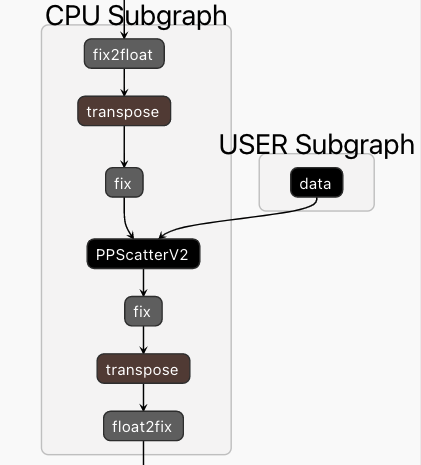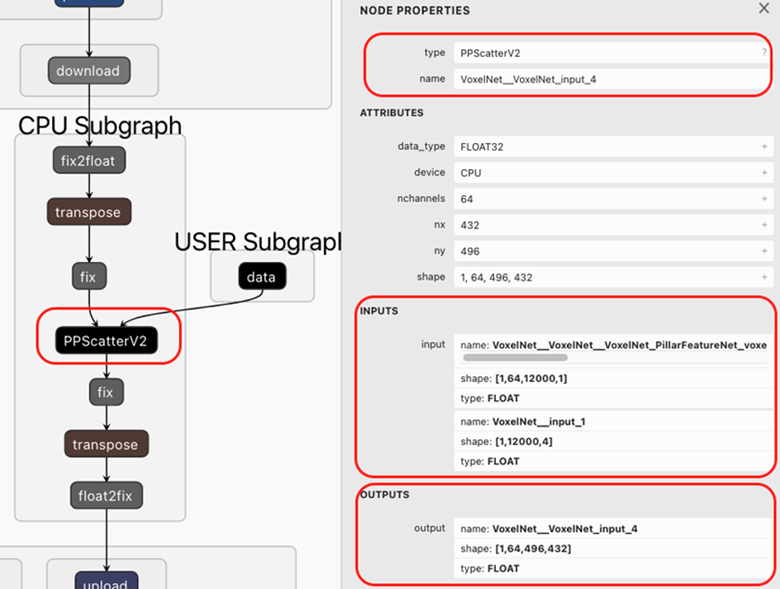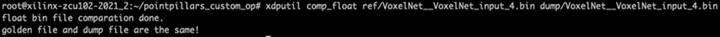# Custom OP Registration - 3.5 English

## Vitis AI User Guide (UG1414)

Document ID
UG1414
Release Date
2023-06-29
Version
3.5 English

Before custom op registration, you can use the latest Netron program to check the compiled model. From the following graph, PPScatter is assigned to the CPU. You have to implement and register PPScatter OP.

Figure 1. PPScatter OP in CPU Subgraph## Steps

1. Use Netron to open the compiled model and find the custom OP in CPU subgraph with op information.
Figure 2. The inputs and outputs of PPScatter OpFrom the previous model structure image, you can find the OP type is PPScatterV2, which is the name of the custom OP that needs to be created.

You can also use xdputil to check the OP's detailed information. Run the following command to check the custom_layer OP.
``xdputil xmodel pointpillars_custom_op.xmodel --op VoxelNet__VoxelNet_input_4``
2. Write your own implementation of this op.

Custom OP registration supports both C++ and Python. The following shows how to implement the OP in C++. For the OP Python implementation, refer to Vitis-AI/examples/custom_operator/pytorch_example/op_registration/python/

Note: There is README.md file in Vitis-AI/examples/custom_operator/op_add directory which illustrates the detailed steps on how to implement the custom op. You can refer to it on how to implement and register the custom OP.
1. Create the my_PPScatter_op.cpp source file and put it under new folder op_PPScatter.

You can also copy one existed op and renamed to your op, as shown below. Then, rename my_tanh_op.cpp to my_PPScatter_op.cpp.

``cp - r Vitis-AI/src/vai_library/cpu_task/examples/op_tanh/  op_PPScatter ``
2. Create the Makefile.
``````
OUTPUT_DIR = \$(PWD)

all: \$(OUTPUT_DIR) \$(OUTPUT_DIR)/libvart_op_imp_PPScatterV2.so

\$(OUTPUT_DIR):
mkdir -p \$@

\$(OUTPUT_DIR)/my_PPScatter_op.o: my_PPScatter_op.cpp
\$(CXX) -std=c++17 -fPIC -c -o \$@ -I. -I=/install/Debug/include -Wall -U_FORTIFY_SOURCE -D_FORTIFY_SOURCE=0 \$<

\$(OUTPUT_DIR)/libvart_op_imp_PPScatterV2.so: \$(OUTPUT_DIR)/my_PPScatter_op.o
\$(CXX) -Wl,--no-undefined -shared -o \$@ \$+ -L=/install/Debug/lib -lglog -lvitis_ai_library-runner_helper -lvart-runner -lxir``````
3. Write the implementation of the OP.

In my_PPScatter_op.cpp, use the construct function to initialize some variable; in this example, there is no variable need be initialized.

In the calculate() function, implementation your own logic. The logic is mainly getting input data from “inputs” variable, calculating the logic, writing output data to the “output” variable.

The code of my_PPScatter_op.cpp is shown below.
``````
#include <vart/op_imp.h>

class MyPPScatterOp {
public:
MyPPScatterOp(const xir::Op* op1, xir::Attrs* attrs) : op{op1} {
// op and attrs is not in use.
}

int calculate(vart::simple_tensor_buffer_t output,
std::vector<vart::simple_tensor_buffer_t<float>> inputs) {
CHECK_EQ(inputs.size(), 2);
auto input_data_shape = inputs.tensor->get_shape();
auto input_coord_shape = inputs.tensor->get_shape();
auto output_shape = output.tensor->get_shape();
CHECK_EQ(input_data_shape.size(), 4); // 1 12000 1 64 --> 1 64 12000 1
CHECK_EQ(input_coord_shape.size(), 3); // 1 12000 4
CHECK_EQ(output_shape.size(), 4); // 1 496 432 64 ---> 1 64 496 432

auto coord_numbers = input_coord_shape;
auto coord_channel = input_coord_shape;
CHECK_EQ(coord_numbers, input_data_shape);

auto batch = output_shape;
auto height = output_shape;
auto width = output_shape;
auto channel = output_shape;
CHECK_EQ(input_data_shape, batch);
CHECK_EQ(channel, input_data_shape);

auto output_idx = 0;
auto input_idx = 0;
auto x_idx = 0;

memset(output.data, 0, output_shape*output_shape*output_shape*output_shape*sizeof(float));

for (auto n = 0; n < coord_numbers; n++) {
auto x = (int)inputs.data[x_idx + 3];
auto y = (int)inputs.data[x_idx + 2];
if (x < 0) break; // stop copy data when coord x == -1 .
for(int i=0; i < channel; i++) {
output_idx =i*height*width + y*width+x;
input_idx = n+i*coord_numbers;
output.data[output_idx] = inputs.data[ input_idx ];
}
x_idx += coord_channel;
}
return 0;
}

public:
const xir::Op* const op;
};

DEF_XIR_OP_IMP(MyPPScatterOp)
``````
4. Build the library. The target directory is \$(HOME)/build/custom_op/ .  You can modify the path in Makefile.

Running make with your Makefile, you’ll see the custom defined op library is generated in \$(HOME)/build/custom_op/, file name is libvart_op_imp_PPScatterV2.so.

5. Copy the libvart_op_imp_PPScatterV2.so to /usr/lib on the target.
3. Verify the Op on the target.
1. Use run_op command in xdputil to test the op, as shown below.
``xdputil run_op pointpillars_op.xmodel VoxelNet__VoxelNet_input_4 -r ref -d dump``

Before running the above command, prepare the reference inputs of the op. After you run the command successfully, VoxelNet__VoxelNet_input_4.bin file will be generated.

2. Compare the output with the golden file. The command is shown below.
`` xdputil comp_float ref/VoxelNet__VoxelNet_input_4.bin dump/VoxelNet__VoxelNet_input_4.bin``

If the OP implementation is successful, you will see the following result: